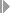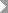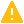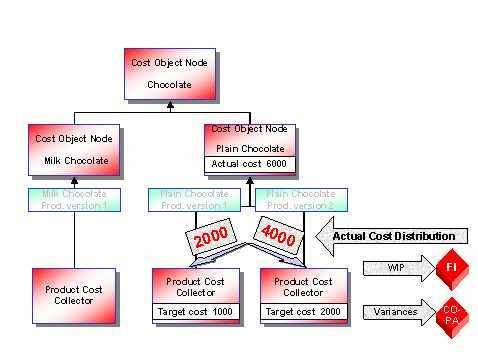Show TOC

###Period-End Closing for Cost Object Hierarchies With Distribution#### Prerequisites

Read the following sections:

You specify the following parameters in Customizing for Product Cost Controlling underCost Object ControllingProduct Cost by PeriodCost Object HierarchiesDefine Cost Object Categories for Cost Object Hierarchies:

• Whether distribution is active

• Whether overhead allocation is allowed

• Whether variances are calculated at the level of the cost object hierarchy

• Whether variance calculation and settlement is made for all orders (product cost collectors or manufacturing orders) for a material through the cost object hierarchy, or whether individual orders can be processed separately.Caution

If you update quantities and values separately in Materials Management (MM), make sure that all quantity movements are valuated before you run the period-end closing transactions.

End of the caution.

#### Process Flow

You can use the Schedule Manager in the period-end closing process of Product Cost by Period . The Schedule Manager simplifies the period-end closing process. In Product Cost by Period , you can use the multilevel worklist of the Schedule Manager . The multilevel worklist can significantly improve performance and facilitate error correction.

Multilevel worklists enable you to do such things as:

• Display the objects in a processing step sequence that have errorsNote

Each function in the period-end closing process is regarded as a separate processing step. A group of processing steps that are performed one after the other is called a processing step sequence .

End of the note.
• Eliminate the cause of the error, or set a processing status that instructs the system to skip that particular processing step

• Instruct the system to perform a particular processing step sequence again (once you have corrected the errors or set processing statuses that control how objects are further processed)

The system then only processes the objects that had errors and the objects whose processing was forced manually.

The following section contains information on using the Schedule Manager in connection with a multilevel worklist: Starting Transactions/Reports Online, Scheduling Jobs and Job Chains, Worklist

The following functions are available in the period-end closing process for cost object hierarchies:

If you have activated distribution in Customizing under Define Cost Object Categories for Cost Object Hierarchies , you distribute the actual costs (Distribution of Cost Object Hierarchy Costs).

When you perform distribution, the system determine the equivalences between the assigned orders by calculating the target costs for each cost element (or for each cost element, origin, and period) using the cost estimate on which the target cost version is based and the quantity delivered to inventory during the period. Equivalences for actual cost distribution can be calculated on the basis of target cost versions 0, 1, or 3.

The system then distributes the actual costs to the assigned single objects on the basis of these equivalences. Actual costs that cannot be assigned to a cost element on the assigned single object are assigned to a cost element group selected through the target cost version.

The distributed actual costs are updated to the orders (such as product cost collectors) assigned to the cost object hierarchy. These costs are included in the period-end closing process of the assigned orders.

#### Result

Once you have performed distribution, you perform the functions of the period-end closing process that follow distribution (such as WIP calculation, variance calculation, and settlement) with reference to the orders assigned to the cost object hierarchy. You can still perform these steps in the period-end closing process for the cost object hierarchy.

Once you have distributed the actual costs charged to the cost object nodes, you normally perform overhead calculation at the level of the single objects (such as product cost collectors) assigned to the cost object hierarchy. In this case you should not turn on the Overhead indicator in Customizing underProduct Cost by PeriodCost Object HierarchiesDefine Cost Object Categories for Cost Object Hierarchies. This prevents entry of a costing sheet and an overhead key for the cost object nodes of the cost object hierarchy. After distribution, you calculate the overhead for the orders assigned to the lowest cost object nodes.

#### Cost Object Hierarchy with Distribution: WIP Calculation, Variance Calculation, and Settlement( )

Work in process is calculated at the level of the orders (such as product cost collectors or manufacturing orders) assigned to the cost object hierarchy. In joint production, it is not possible to calculate WIP at target costs (see above). You can start WIP calculation through the cost object hierarchy. Work in process is calculated for all orders that are assigned to a cost object. In collective processing, you can calculate work in process for all orders in a plant, regardless of whether they are assigned to a cost object hierarchy.

You cannot calculate work in process for the cost object nodes themselves.

The calculation of WIP at target costs includes the quantities confirmed at the operations or reporting points. The actual costs distributed to the assigned orders are not included in WIP calculation.

If distribution is active, you calculate variances for the orders assigned to the cost object hierarchy. You must still start variance calculation by calculating the variances for the cost object hierarchy as a whole.

If you have distributed the actual costs, the amount to be settled is calculated with the following formula:

Amount to be settled = Charged actual costs of period – Credit posting for goods receipt – Work in process

The amount to be settled is therefore the actual cost less the work in process. If distribution is active, settlement takes place at the level of the assigned orders.

If you have activated distribution in Customizing under Define Cost Object Categories for Cost Object Hierarchies , you don’t need to specify a settlement profile for the cost object nodes of the cost object hierarchy because settlement is performed through the orders assigned to the cost object hierarchy. You can settle the orders assigned to the cost object hierarchy at the level of the cost object hierarchy.

#### Result

You can see the data calculated by the period-end closing transactions in the Product Cost Controlling Information System .

In the Product Cost Controlling Information System you can choose between displaying the data entered on the cost object node (data entry object) and displaying the data summarized on a cost object node (summarization object). To display the summarized data, first execute a data collection run.

For more information, refer to the section Information System for Product Cost by Period and the sections following in the structure.